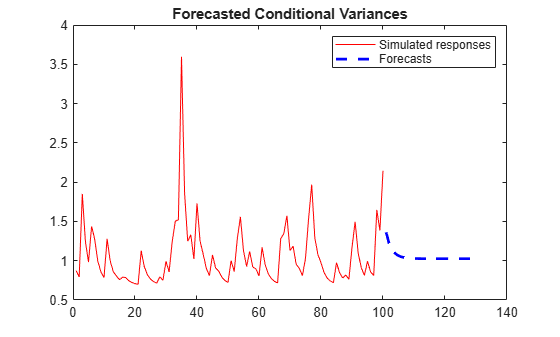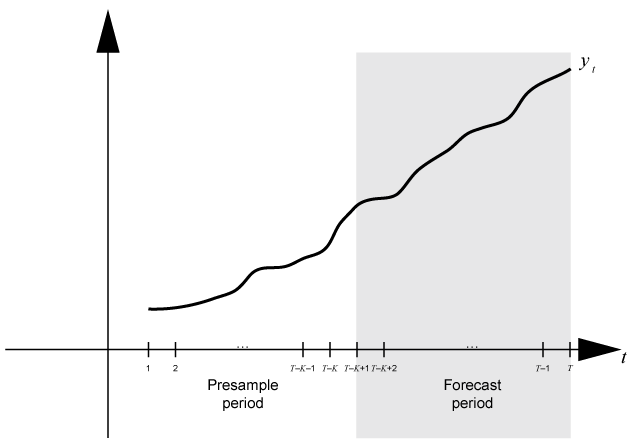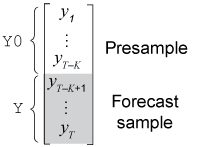# forecast

Forecast conditional variances from conditional variance models

## Syntax

``V = forecast(Mdl,numperiods,Y0)``
``Tbl2 = forecast(Mdl,numperiods,Tbl1)``
``[___] = forecast(___,Name,Value)``

## Description

example

````V = forecast(Mdl,numperiods,Y0)` returns a numeric array containing paths of minimum mean squared error (MMSE), consecutive forecasted conditional variances `V` of the fully specified, univariate conditional variance model `Mdl`, over a `numperiods` forecast horizon. The model `Mdl` can be a `garch`, `egarch`, or `gjr` model object. The forecasts represent the continuation of the presample data in the numeric array `Y0`. (since R2019a)```

example

````Tbl2 = forecast(Mdl,numperiods,Tbl1)` returns the table or timetable `Tbl2` containing the paths of MMSE conditional variance variable forecasts of the model `Mdl` over a `numperiods` forecast horizon. `forecast` uses the table or timetable of presample data `Tbl1` to initialize the response series. (since R2023a)To initialize the forecast, `forecast` selects the response variable named in `Mdl.SeriesName` or the sole variable in `Tbl1`. To select a different response variable in `Tbl1` to initialize the forecasts, use the `PresampleResponseVariable` name-value argument.```

example

````[___] = forecast(___,Name,Value)` specifies options using one or more name-value arguments in addition to any of the input argument combinations in previous syntaxes. `forecast` returns the output argument combination for the corresponding input arguments. For example, `forecast(Mdl,10,Y0,V0=v0)` initializes the conditional variances for the forecast using the presample data in `v0`.```

## Examples

collapse all

Forecast the conditional variance of simulated data over a 30-period horizon. Supply a vector of presample response data.

Simulate 100 observations from a GARCH(1,1) model with known parameters.

```Mdl = garch(Constant=0.02,GARCH=0.8,ARCH=0.1); rng("default") % For reproducibility [v,y] = simulate(Mdl,100);```

Forecast the conditional variances over a 30-period horizon. Specify the simulated response data. Plot the forecasts.

```vF = forecast(Mdl,30,y); figure plot(v,"-b") hold on plot(101:130,vF,"r--",LineWidth=2); title("Forecasted Conditional Variances") legend("Simulated presample","Forecasts") hold off```Forecasts converge asymptotically to the unconditional innovation variance.

Forecast the conditional variance of simulated data over a 30-period horizon.

Simulate 100 observations from an EGARCH(1,1) model with known parameters.

```Mdl = egarch(Constant=0.01,GARCH=0.6,ARCH=0.2, ... Leverage=-0.2); rng("default") % For reproducibility [v,y] = simulate(Mdl,100);```

Forecast the conditional variance over a 30-period horizon. Specify the simulated data as presample responses. Plot the forecasts.

```VF1 = forecast(Mdl,30,y); figure plot(v,"r-") hold on plot(101:130,VF1,"b--",LineWidth=2); title("Forecasted Conditional Variances") legend("Simulated responses","Forecasts") hold off```Forecast the conditional variance of simulated data over a 30-period horizon.

Simulate 100 observations from a GJR(1,1) model with known parameters.

```Mdl = gjr(Constant=0.01,GARCH=0.6,ARCH=0.2, ... Leverage=0.2); rng("default") % For reproducibility [v,y] = simulate(Mdl,100);```

Forecast the conditional variances over a 30-period horizon. Specify the simulated presample responses. Plot the forecasts.

```vF = forecast(Mdl,30,y); figure plot(v,"r") hold on plot(101:130,vF,'b--',LineWidth=2); title("Forecasted Conditional Variances") legend("Observed","Forecasts") hold off```Since R2023a

Forecast the conditional variance of the NASDAQ Composite Index returns from fitted GARCH(1,1), EGARCH(1,1) and GJR(1,1) models.

Load the NASDAQ data included with the toolbox. Convert the index to returns. Plot the returns.

```load Data_EquityIdx DTTRet = price2ret(DataTimeTable); DTTRet.Interval = []; T = height(DTTRet); meanRet = mean(DTTRet.NASDAQ)```
```meanRet = 4.7771e-04 ```
```figure plot(DTTRet.Time,100*DTTRet.NASDAQ); hold on yline(100*meanRet,'--r') title("Daily NASDAQ Returns"); xlabel("Date"); ylabel("Return (%)");```The variance of the series seems to change. This change is an indication of volatility clustering. The conditional mean model offset is very close to zero.

Fit GARCH(1,1), EGARCH(1,1), and GJR(1,1) models to the data. By default, the software sets the conditional mean model offset to zero.

```MdlGARCH = garch(1,1); MdlEGARCH = egarch(1,1); MdlGJR = gjr(1,1); EstMdlGARCH = estimate(MdlGARCH,DTTRet,ResponseVariable="NASDAQ"); ```
``` GARCH(1,1) Conditional Variance Model (Gaussian Distribution): Value StandardError TStatistic PValue __________ _____________ __________ __________ Constant 2.0101e-06 5.4314e-07 3.7008 0.00021491 GARCH{1} 0.8833 0.0084528 104.5 0 ARCH{1} 0.10919 0.007662 14.251 4.4113e-46 ```
`EstMdlEGARCH = estimate(MdlEGARCH,DTTRet,ResponseVariable="NASDAQ"); `
``` EGARCH(1,1) Conditional Variance Model (Gaussian Distribution): Value StandardError TStatistic PValue _________ _____________ __________ __________ Constant -0.13494 0.022096 -6.1073 1.0135e-09 GARCH{1} 0.98389 0.0024225 406.15 0 ARCH{1} 0.19964 0.013964 14.297 2.2804e-46 Leverage{1} -0.060242 0.0056459 -10.67 1.4067e-26 ```
`EstMdlGJR = estimate(MdlGJR,DTTRet,ResponseVariable="NASDAQ");`
``` GJR(1,1) Conditional Variance Model (Gaussian Distribution): Value StandardError TStatistic PValue __________ _____________ __________ __________ Constant 2.4567e-06 5.6828e-07 4.3231 1.5386e-05 GARCH{1} 0.88144 0.009478 92.998 0 ARCH{1} 0.06394 0.009177 6.9674 3.2294e-12 Leverage{1} 0.088908 0.0099025 8.9784 2.7469e-19 ```

Forecast the conditional variance for 14 days using the fitted models. Use the observed returns as presample innovations for the forecasts.

```fh = 14; DTTVFGARCH = forecast(EstMdlGARCH,fh,DTTRet, ... PresampleResponseVariable="NASDAQ"); DTTVFEGARCH = forecast(EstMdlEGARCH,fh,DTTRet, ... PresampleResponseVariable="NASDAQ"); DTTVFGJR= forecast(EstMdlGJR,fh,DTTRet, ... PresampleResponseVariable="NASDAQ");```

The forecasted conditional variance variables are called `Y_Variance` in each returned timetable.

Plot the forecasts along with the conditional variances inferred from the data.

```DTTVGARCH = infer(EstMdlGARCH,DTTRet,ResponseVariable="NASDAQ"); DTTVEGARCH = infer(EstMdlEGARCH,DTTRet,ResponseVariable="NASDAQ"); DTTVGJR = infer(EstMdlGJR,DTTRet,ResponseVariable="NASDAQ"); figure tiledlayout(3,1) nexttile plot(DTTRet.Time(end-100:end),DTTVGARCH.Y_Variance(end-100:end), ... "r",DTTVFGARCH.Time,DTTVFGARCH.Y_Variance,"b--") legend("Inferred","Forecast",Location="northeast") title("GARCH(1,1) Conditional Variances") nexttile plot(DTTRet.Time(end-100:end),DTTVEGARCH.Y_Variance(end-100:end),"r", ... DTTVFEGARCH.Time,DTTVFEGARCH.Y_Variance,"b--") legend("Inferred","Forecast",Location="northeast") title("EGARCH(1,1) Conditional Variances") nexttile plot(DTTRet.Time(end-100:end),DTTVGJR.Y_Variance(end-100:end),"r", ... DTTVFGJR.Time,DTTVFGJR.Y_Variance,'b--') legend("Inferred","Forecast",Location="northeast") title("GJR(1,1) Conditional Variances")```Plot conditional variance forecasts for the next 1000 days after the sample.

```fh = 1000; DTTVF1000GARCH = forecast(EstMdlGARCH,fh,DTTRet, ... PresampleResponseVariable="NASDAQ"); DTTVF1000EGARCH = forecast(EstMdlEGARCH,fh,DTTRet, ... PresampleResponseVariable="NASDAQ"); DTTVF1000GJR= forecast(EstMdlGJR,fh,DTTRet, ... PresampleResponseVariable="NASDAQ"); figure plot(DTTVF1000GARCH.Time,DTTVF1000GARCH.Y_Variance,'b',... DTTVF1000EGARCH.Time,DTTVF1000EGARCH.Y_Variance,'r',... DTTVF1000GJR.Time,DTTVF1000GJR.Y_Variance,'k') legend("GARCH Forecast","EGARCH Foecast","GJR Forecast",Location="northeast") title("Long-Run Conditional Variance Forecast")```The forecasts converge asymptotically to the unconditional variances of their respective processes.

## Input Arguments

collapse all

Conditional variance model without any unknown parameters, specified as a `garch`, `egarch`, or `gjr` model object.

`Mdl` cannot contain any properties that have `NaN` value.

Forecast horizon, or the number of time points in the forecast period, specified as a positive integer.

Data Types: `double`

Since R2019a

Presample response data yt used to infer presample innovations εt, and whose conditional variance process σt2 is forecasted, specified as a `numpreobs`-by-1 numeric column vector or a `numpreobs`-by-`numpaths` numeric matrix.

`numpreobs` is the number of presample observations.

`Y0` can represent a mean 0 presample innovations series with a variance process characterized by the conditional variance model `Mdl`. `Y0` can also represent a presample innovations series plus an offset (stored in `Mdl.Offset`). For more details, see Algorithms.

Each row is a presample observation, and measurements in each row, among all pages, occur simultaneously. The last row contains the latest presample observation. `numpreobs` must be at least `Mdl.Q` to initialize the conditional variance model. If `numpreobs` > `Mdl.Q`, `forecast` uses only the latest `Mdl.Q` rows. For more details, see Time Base Partitions for Forecasting.

Columns of `Y0` correspond to separate, independent paths.

• If `Y0` is a column vector, it represents a single path of the response series. `forecast` applies it to each forecasted path. In this case, all forecast paths `Y` derive from the same initial responses.

• If `Y0` is a matrix, each column represents a presample path of the response series. `numpaths` is the maximum among the second dimensions of the specified presample observation matrices `Y0` and `V0`.

Data Types: `double`

Since R2023a

Presample data containing the response variable yt and, optionally, the conditional variance variable σt2 used to initialize the model for the forecast, specified as a table or timetable, the same type as `Tbl1`, with `numprevars` variables and `numpreobs` rows. You can select a response variable or conditional variance variable from `Tbl1` by using the `PresampleResponseVariable` or `PresampleVarianceVariable` name-value argument, respectively.

Each selected variable is single path (`numpreobs`-by-1 vector) or multiple paths (`numpreobs`-by-`numpaths` matrix) of presample response or conditional variance data. Each row is a presample observation, and measurements in each row occur simultaneously. `numpreobs` must be one of the following values:

• `Mdl.Q` when `Tbl1` provides only presample responses

• `max([Mdl.P Mdl.Q])` when `Tbl1` also provides presample conditional variances

If you supply more rows than necessary, `forecast` uses the latest required number of observations only.

If `Tbl1` is a timetable, all the following conditions must be true:

• `Tbl1` must represent a sample with a regular datetime time step (see `isregular`).

• The datetime vector of sample timestamps `Tbl1.Time` must be ascending or descending.

If `Tbl1` is a table, the last row contains the latest presample observation.

Although `forecast` requires presample response data, `forecast` sets default presample conditional variance data in one of the following ways:

• If `numpreobs````max([Mdl.P Mdl.Q]) + Mdl.P```, `forecast` infers presample conditional variances from the presample response data (see `infer`).

• Otherwise:

• If `Mdl` is a GARCH(P,Q) or GJR(P,Q) model, `forecast` sets all required conditional variances to the unconditional variance of the conditional variance process.

• If `Mdl` is a EGARCH(P,Q) model, `forecast` sets all required conditional variances to the exponentiated, unconditional mean of the logarithm of the EGARCH(P,Q) variance process.

### Name-Value Arguments

Specify optional pairs of arguments as `Name1=Value1,...,NameN=ValueN`, where `Name` is the argument name and `Value` is the corresponding value. Name-value arguments must appear after other arguments, but the order of the pairs does not matter.

Before R2021a, use commas to separate each name and value, and enclose `Name` in quotes.

Example: `forecast(Mdl,10,Y0,V0=[1 0.5;1 0.5])` specifies two different presample paths of conditional variances.

Presample conditional variances σt2 used to initialize the conditional variance model, specified as a `numpreobs`-by-1 positive column vector or `numpreobs`-by-`numpaths`positive matrix with positive entries and `numpaths` columns. Use `V0` only when you supply the numeric array of presample response data `Y0`.

Rows of `V0` correspond to periods in the presample, and the last row contains the latest presample conditional variance.

• For GARCH(P,Q) and GJR(P,Q) models, `numpreobs` must be at least `Mdl.P` to initialize the variance equation.

• For EGARCH(P,Q) models, `numpreobs` must be at least `max([Mdl.P Mdl.Q])` to initialize the variance equation.

If `numpreobs` exceeds the minimum number, `forecast` uses only the latest observations.

Columns of `V0` correspond to separate, independent paths.

• If `V0` is a column vector, `forecast` applies it to each forecasted path. In this case, the conditional variance model of all forecast paths `V` derives from the same initial conditional variances.

• If `V0` is a matrix, it must have `numpaths` columns, the same number of columns as `Y0`.

`forecast` sets default presample conditional variance data in one of the following ways:

• If the number of rows of `Y0` `numpreobs````max([Mdl.P Mdl.Q]) + Mdl.P```, `forecast` infers `V0` from `Y0` (see `infer`).

• Otherwise:

• If `Mdl` is a GARCH(P,Q) or GJR(P,Q) model, `forecast` sets all required conditional variances to the unconditional variance of the conditional variance process.

• If `Mdl` is a EGARCH(P,Q) model, `forecast` sets all required conditional variances to the exponentiated, unconditional mean of the logarithm of the EGARCH(P,Q) variance process.

Data Types: `double`

Since R2023a

Variable of `Tbl1` containing presample response paths yt, specified as one of the following data types:

• String scalar or character vector containing a variable name in `Tbl1.Properties.VariableNames`

• Variable index (integer) to select from `Tbl1.Properties.VariableNames`

• A length `numprevars` logical vector, where ```PresampleResponseVariable(j) = true``` selects variable `j` from `Tbl1.Properties.VariableNames`, and `sum(PresampleResponseVariable)` is `1`

The selected variable must be a numeric matrix and cannot contain missing values (`NaN`).

If `Tbl1` has one variable, the default specifies that variable. Otherwise, the default matches the variable to name in `Mdl.SeriesName`.

Example: `PresampleResponseVariable="StockRate"`

Example: ```PresampleResponseVariable=[false false true false]``` or `PresampleResponseVariable=3` selects the third table variable as the presample response variable.

Data Types: `double` | `logical` | `char` | `cell` | `string`

Since R2023a

Variable of `Tbl1` containing presample conditional variance paths σt2, specified as one of the following data types:

• String scalar or character vector containing a variable name in `Tbl1.Properties.VariableNames`

• Variable index (integer) to select from `Tbl1.Properties.VariableNames`

• A length `numprevars` logical vector, where ```PresampleVarianceVariable(j) = true``` selects variable `j` from `Tbl1.Properties.VariableNames`, and `sum(PresampleVarianceVariable)` is `1`

The selected variable must be a numeric vector and cannot contain missing values (`NaN`).

To use presample conditional variance data in `Tbl1`, you must specify `PresampleVarianceVariable`.

Example: `PresampleVarianceVariable="StockRateVar"`

Example: ```PresampleVarianceVariable=[false false true false]``` or `PresampleVarianceVariable=3` selects the third table variable as the presample conditional variance variable.

Data Types: `double` | `logical` | `char` | `cell` | `string`

Note

• `NaN` values in nuneric presample data sets `Y0` and `V0` indicate missing data. `forecast` removes missing data from the presample data sets following this procedure:

1. `forecast` horizontally concatenates `Y0` and `V0` such that the latest observations occur simultaneously. The result can be a jagged array because the presample data sets can have a different number of rows. In this case, `forecast` prepads variables with an appropriate amount of zeros to form a matrix.

2. `forecast` applies list-wise deletion to the combined presample matrix by removing all rows containing at least one `NaN`.

3. `forecast` extracts the processed presample data sets from the result of step 2, and removes all prepadded zeros.

List-wise deletion reduces the sample size and can create irregular time series.

• For numeric data inputs, `forecast` assumes that you synchronize the presample data such that the latest observations occur simultaneously.

• `forecast` issues an error when any table or timetable input contains missing values.

## Output Arguments

collapse all

Paths of MMSE forecasts of conditional variances σt2 of future model innovations εt, returned as a `numperiods`-by-1 numeric column vector or a `numperiods`-by-`numpaths` numeric matrix. `forecast` returns `V` only when you supply the input `Y0`.

`V` represents a continuation of `V0` (`V(1,:)` occurs in the next time point after `V0(end,:)`).

`V(j,k)` contains the `j`-period-ahead forecasted conditional variance of path `k`.

`forecast` determines `numpaths` from the number of columns in the presample data sets `Y0` and `V0`. For details, see Algorithms. If each presample data set has one column, then `V` is a column vector.

Since R2023a

Paths of MMSE forecasts of conditional variances σt2 of future model innovations εt, returned as a table or timetable, the same data type as `Tbl1`. `forecast` returns `Tbl2` only when you supply the input `Tbl1`.

`Tbl2` contains a variable for all forecasted conditional variance paths, which are in a `numperiods`-by-`numpaths` numeric matrix, with rows representing periods in the forecast horizon and columns representing independent paths, each corresponding to the input presample response and conditional variance paths in `Tbl1`. `forecast` names the forecasted conditional variance variable in `Tbl2` `responseName_Variance`, where `responseName` is `Mdl.SeriesName`. For example, if `Mdl.SeriesName` is `StockReturns`, `Tbl2` contains a variable for the corresponding forecasted conditional variance paths with the name `StockReturns_Variance`.

`Tbl2.responseName_Variance` represents a continuation of the presample conditional variance process, either supplied by `Tbl1` or set by default (`Tbl2.responseName_Variance(1,:)` occurs in the next time point, with respect to the periodicity `Tbl1`, after the last presample conditional variance).

`Tbl2.responseName_Variance(j,k)`contains the `j`-period-ahead forecasted conditional variance of path `k`.

If `Tbl1` is a timetable, the following conditions hold:

• The row order of `Tbl1`, either ascending or descending, matches the row order of `Tbl2`.

• `Tbl2.Time(1)` is the next time after `Tbl1.Time(end)` relative the sampling frequency, and `Tbl2.Time(2:numobs)` are the following times relative to the sampling frequency.

collapse all

### Time Base Partitions for Forecasting

Time base partitions for forecasting are two disjoint, contiguous intervals of the time base; each interval contains time series data for forecasting a dynamic model. The forecast period (forecast horizon) is a `numperiods` length partition at the end of the time base during which `forecast` generates forecasts `V` from the dynamic model `Mdl`. The presample period is the entire partition occurring before the forecast period. `forecast` can require observed responses (or innovations) `Y0` or conditional variances `V0` in the presample period to initialize the dynamic model for forecasting. The model structure determines the types and amounts of required presample observations.

A common practice is to fit a dynamic model to a portion of the data set, then validate the predictability of the model by comparing its forecasts to observed responses. During forecasting, the presample period contains the data to which the model is fit, and the forecast period contains the holdout sample for validation. Suppose that yt is an observed response series. Consider forecasting conditional variances from a dynamic model of yt `numperiods` = K periods. Suppose that the dynamic model is fit to the data in the interval [1,TK] (for more details, see `estimate`). This figure shows the time base partitions for forecasting.For example, to generate forecasts `Y` from a GARCH(0,2) model, `forecast` requires presample responses (innovations) `Y0` = ${\left[\begin{array}{cc}{y}_{T-K-1}& {y}_{T-K}\end{array}\right]}^{\prime }$ to initialize the model. The 1-period-ahead forecast requires both observations, whereas the 2-periods-ahead forecast requires yTK and the 1-period-ahead forecast `V(1)`. `forecast` generates all other forecasts by substituting previous forecasts for lagged responses in the model.

Dynamic models containing a GARCH component can require presample conditional variances. Given enough presample responses, `forecast` infers the required presample conditional variances. This figure shows the arrays of required observations for this case, with corresponding input and output arguments.## Algorithms

• If the conditional variance model `Mdl` has an offset (`Mdl.Offset`), `forecast` subtracts it from the specified presample responses to obtain presample innovations. Subsequently, `forecast` uses to initialize the conditional variance model for forecasting.

• `forecast` sets the number of sample paths to forecast `numpaths` to the maximum number of columns among the specified presample response and conditional variance data sets. All presample data sets must have either `numpaths` > 1 columns or one column. Otherwise, `forecast` issues an error. For example, if `Y0` has five columns, representing five paths, then `V0` can either have five columns or one column. If `V0` has one column, then `forecast` applies `V0` to each path.

 Bollerslev, T. “Generalized Autoregressive Conditional Heteroskedasticity.” Journal of Econometrics. Vol. 31, 1986, pp. 307–327.

 Bollerslev, T. “A Conditionally Heteroskedastic Time Series Model for Speculative Prices and Rates of Return.” The Review of Economics and Statistics. Vol. 69, 1987, pp. 542–547.

 Box, G. E. P., G. M. Jenkins, and G. C. Reinsel. Time Series Analysis: Forecasting and Control. 3rd ed. Englewood Cliffs, NJ: Prentice Hall, 1994.

 Enders, W. Applied Econometric Time Series. Hoboken, NJ: John Wiley & Sons, 1995.

 Engle, R. F. “Autoregressive Conditional Heteroskedasticity with Estimates of the Variance of United Kingdom Inflation.” Econometrica. Vol. 50, 1982, pp. 987–1007.

 Glosten, L. R., R. Jagannathan, and D. E. Runkle. “On the Relation between the Expected Value and the Volatility of the Nominal Excess Return on Stocks.” The Journal of Finance. Vol. 48, No. 5, 1993, pp. 1779–1801.

 Hamilton, J. D. Time Series Analysis. Princeton, NJ: Princeton University Press, 1994.

 Nelson, D. B. “Conditional Heteroskedasticity in Asset Returns: A New Approach.” Econometrica. Vol. 59, 1991, pp. 347–370.